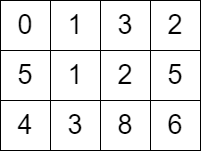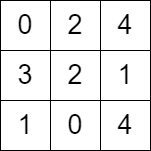2577. Minimum Time to Visit a Cell In a Grid
Hard
334
6

You are given a `m x n` matrix `grid` consisting of non-negative integers where `grid[row][col]` represents the minimum time required to be able to visit the cell `(row, col)`, which means you can visit the cell `(row, col)` only when the time you visit it is greater than or equal to `grid[row][col]`.

You are standing in the top-left cell of the matrix in the `0th` second, and you must move to any adjacent cell in the four directions: up, down, left, and right. Each move you make takes 1 second.

Return the minimum time required in which you can visit the bottom-right cell of the matrix. If you cannot visit the bottom-right cell, then return `-1`.

Example 1:```Input: grid = [[0,1,3,2],[5,1,2,5],[4,3,8,6]]
Output: 7
Explanation: One of the paths that we can take is the following:
- at t = 0, we are on the cell (0,0).
- at t = 1, we move to the cell (0,1). It is possible because grid <= 1.
- at t = 2, we move to the cell (1,1). It is possible because grid <= 2.
- at t = 3, we move to the cell (1,2). It is possible because grid <= 3.
- at t = 4, we move to the cell (1,1). It is possible because grid <= 4.
- at t = 5, we move to the cell (1,2). It is possible because grid <= 5.
- at t = 6, we move to the cell (1,3). It is possible because grid <= 6.
- at t = 7, we move to the cell (2,3). It is possible because grid <= 7.
The final time is 7. It can be shown that it is the minimum time possible.
```

Example 2:```Input: grid = [[0,2,4],[3,2,1],[1,0,4]]
Output: -1
Explanation: There is no path from the top left to the bottom-right cell.
```

Constraints:

• `m == grid.length`
• `n == grid[i].length`
• `2 <= m, n <= 1000`
• `4 <= m * n <= 105`
• `0 <= grid[i][j] <= 105`
• `grid == 0`

Accepted
6.2K
Submissions
17.5K
Acceptance Rate
35.4%

Seen this question in a real interview before?
1/4
Yes
No

Discussion (0)

Related Topics